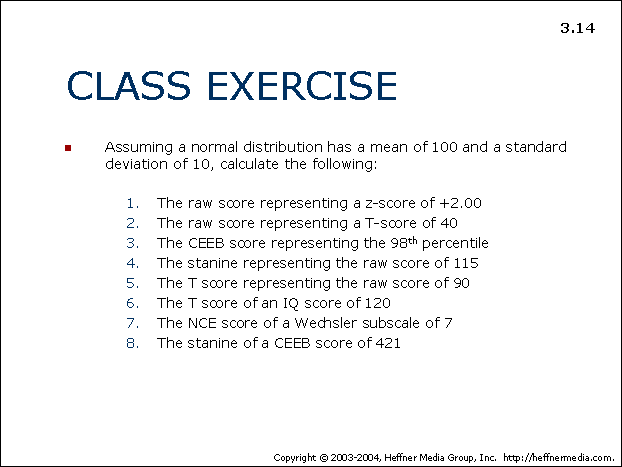# 14: Class Exercise: Calculating## CLASS EXERCISE

• Assuming a normal distribution has a mean of 100 and a standard deviation of 10, calculate the following:
1. The raw score representing a z-score of +2.00
2. The raw score representing a T-score of 40
3. The CEEB score representing the 98th precentile
4. The stanine representing the raw score of 115
5. The T score representing the raw score of 90
6. The T score of an IQ score of 120
7. The NCE score of a Wechsler subscale of 7
8. The stanine of a CEEB score of 421